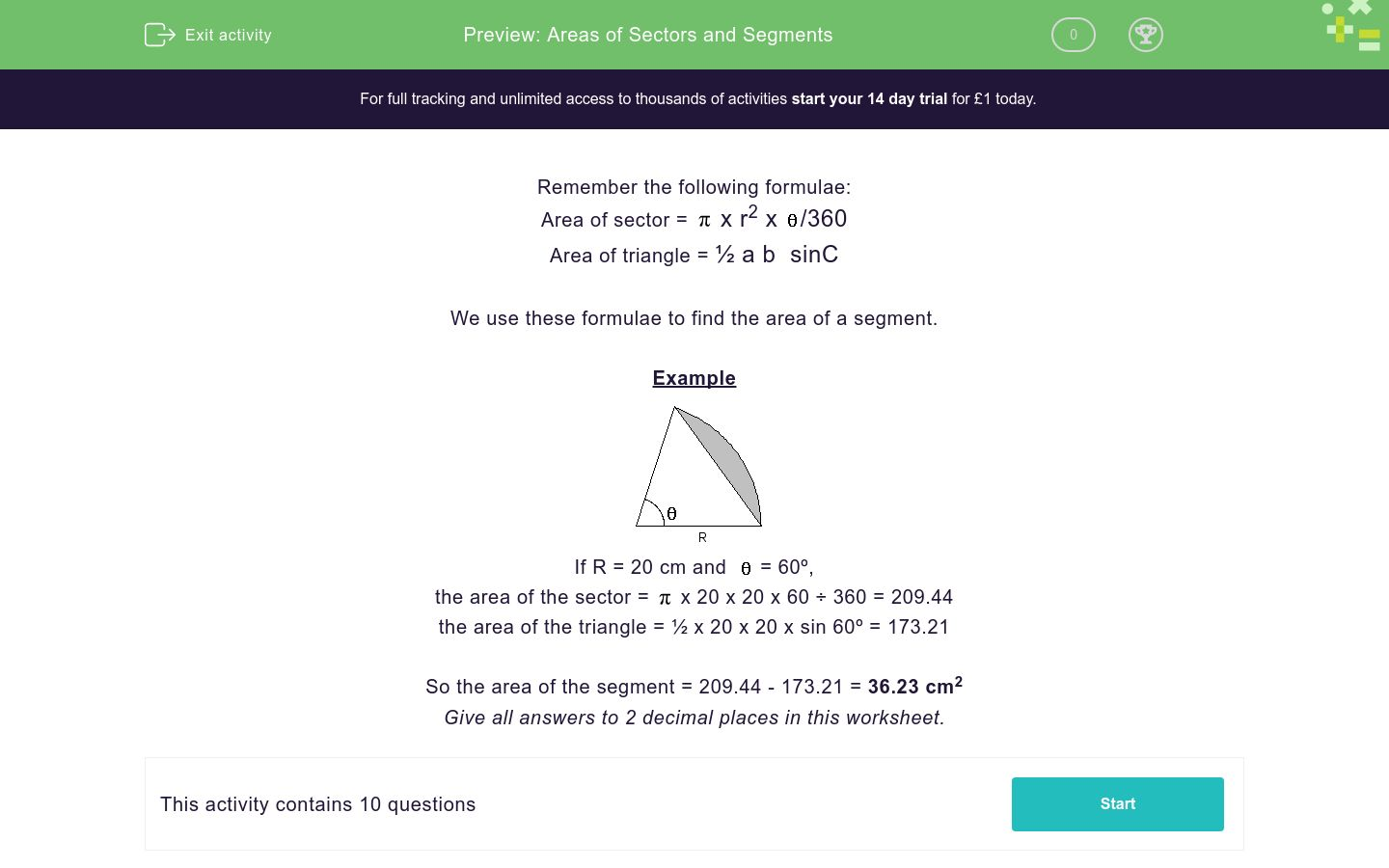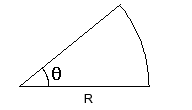# Areas of Sectors and Segments

In this worksheet, students calculate the areas of sectors and segments.Key stage:  KS 4

Curriculum topic:  Geometry and Measures

Curriculum subtopic:  Calculate Arc Lengths, Angles and Areas of Sectors of Circles

Difficulty level:### QUESTION 1 of 10

Remember the following formulae:

Area of sector =x r2 x/360

Area of triangle = ½ a b  sinC

We use these formulae to find the area of a segment.

ExampleIf R = 20 cm and= 60º,

the area of the sector =x 20 x 20 x 60 ÷ 360 = 209.44

the area of the triangle = ½ x 20 x 20 x sin 60º = 173.21

So the area of the segment = 209.44 - 173.21 = 36.23 cm2

Give all answers to 2 decimal places in this worksheet.Calculate the area of the sector.

 Remember, area =x R2 x360

R = 10 cm

θ = 39°

Area = ____ cm2Calculate the area of the segment.

Hint. Find the area of the sector, then the area of the triangle and subtract.

Use ½ab sin C to find the triangle's area.

R = 10 cm

θ = 72°

Area = ____ cm2Calculate the area of the segment.

Hint. Find the area of the sector, then the area of the triangle and subtract.

Use ½ab sin C to find the triangle's area.

R = 12 cm

θ = 41°

Area = ____ cm2Calculate the area of the sector.

 Remember, area =x R2 x360

R = 21 cm

θ = 48°

Area = ____ cm2Calculate the area of the sector.

 Remember, area =x R2 x360

R = 7.5 cm

θ = 35°

Area = ____ cm2Calculate the area of the sector.

 Remember, area =x R2 x360

R = 3.5 cm

θ = 21°

Area = ____ cm2Calculate the area of the segment.

Hint. Find the area of the sector, then the area of the triangle and subtract.

Use ½ab sin C to find the triangle's area.

R = 14 cm

θ = 78°

Area = ____ cm2Calculate the area of the segment.

R = 21.5 cm

θ = 31°

Area = ____ cm2Calculate the area of the segment.

R = 2.5 cm

θ = 22°

Area = ____ cm2Calculate the area of the segment.

R = 10 cm

θ = 44°

Area = ____ cm2

• Question 1Calculate the area of the sector.

 Remember, area =x R2 x360

R = 10 cm

θ = 39°

Area = ____ cm2

CORRECT ANSWER
34.03
• Question 2Calculate the area of the segment.

Hint. Find the area of the sector, then the area of the triangle and subtract.

Use ½ab sin C to find the triangle's area.

R = 10 cm

θ = 72°

Area = ____ cm2

CORRECT ANSWER
15.28
• Question 3Calculate the area of the segment.

Hint. Find the area of the sector, then the area of the triangle and subtract.

Use ½ab sin C to find the triangle's area.

R = 12 cm

θ = 41°

Area = ____ cm2

CORRECT ANSWER
4.29
• Question 4Calculate the area of the sector.

 Remember, area =x R2 x360

R = 21 cm

θ = 48°

Area = ____ cm2

CORRECT ANSWER
184.73
• Question 5Calculate the area of the sector.

 Remember, area =x R2 x360

R = 7.5 cm

θ = 35°

Area = ____ cm2

CORRECT ANSWER
17.18
• Question 6Calculate the area of the sector.

 Remember, area =x R2 x360

R = 3.5 cm

θ = 21°

Area = ____ cm2

CORRECT ANSWER
2.24
• Question 7Calculate the area of the segment.

Hint. Find the area of the sector, then the area of the triangle and subtract.

Use ½ab sin C to find the triangle's area.

R = 14 cm

θ = 78°

Area = ____ cm2

CORRECT ANSWER
37.55
• Question 8Calculate the area of the segment.

R = 21.5 cm

θ = 31°

Area = ____ cm2

CORRECT ANSWER
6.01
• Question 9Calculate the area of the segment.

R = 2.5 cm

θ = 22°

Area = ____ cm2

CORRECT ANSWER
0.03
• Question 10Calculate the area of the segment.

R = 10 cm

θ = 44°

Area = ____ cm2

CORRECT ANSWER
3.66
---- OR ----

Sign up for a £1 trial so you can track and measure your child's progress on this activity.

### What is EdPlace?

We're your National Curriculum aligned online education content provider helping each child succeed in English, maths and science from year 1 to GCSE. With an EdPlace account you’ll be able to track and measure progress, helping each child achieve their best. We build confidence and attainment by personalising each child’s learning at a level that suits them.

Get started#### Similar activities you may like

Start your £1 trial today.
Subscribe from £10/month.